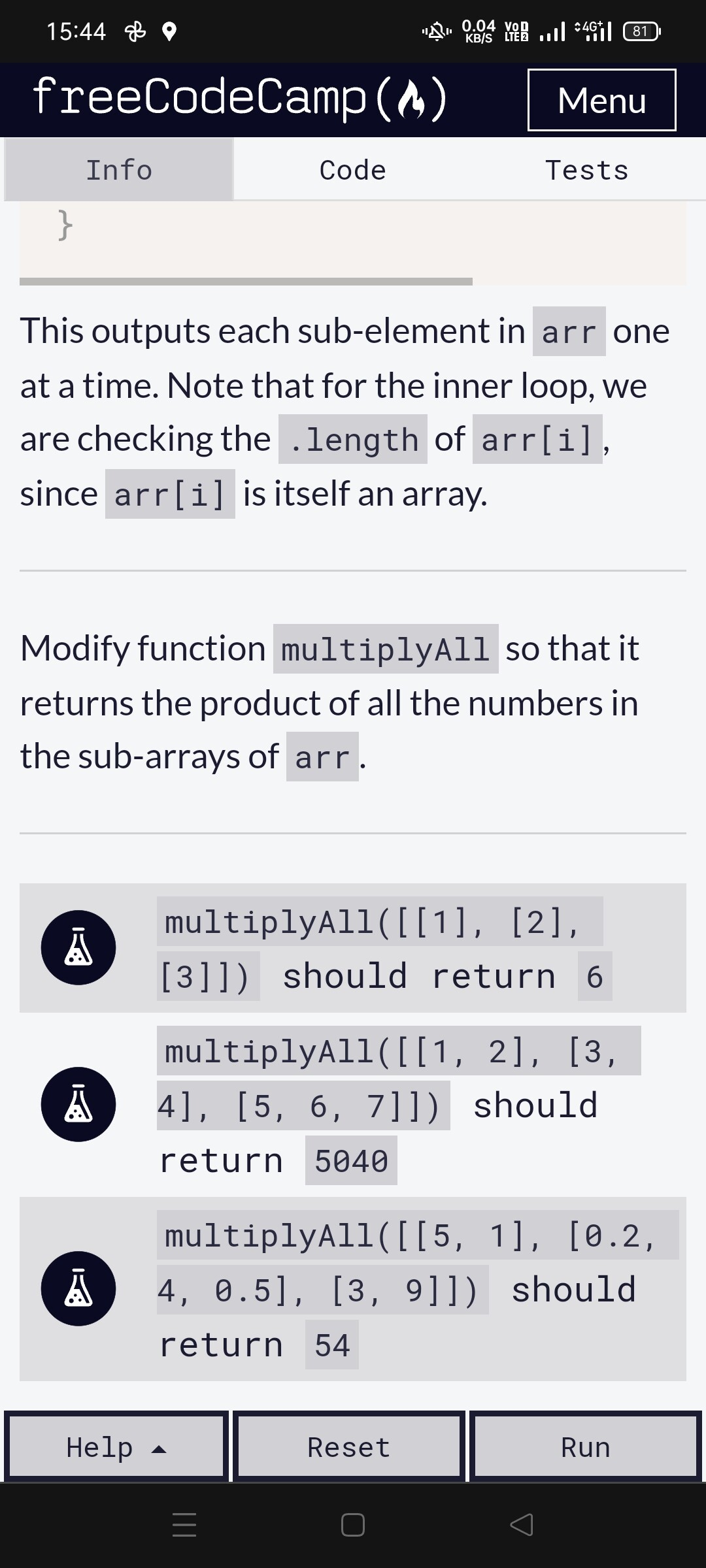# Arithmetic problem with nested array

Tell us what’s happening:
Hello!,

I have been able to solve this exercise, I understand how it works, but I cannot understand this product:

`multiplyAll([[5, 1], [0.2, 4, 0.5], [3, 9]])` should return `54`

``````  **Your code so far**
``````
``````function multiplyAll(arr) {
let product = 1;
// Only change code below this line

for (let i = 0; i < arr.length; i++) {
for (let j = 0; j < arr[i].length; j++) {
product = product * arr[i][j];
}
}
// Only change code above this line
return product;

}

multiplyAll([[1, 2], [3, 4], [5, 6, 7]]);

function multiplyAll(arr) {
let product = 1;
// Only change code below this line

// Only change code above this line
return product;
}

multiplyAll([[1, 2], [3, 4], [5, 6, 7]]);
``````

RESULT= 5040

I can understand well this pair of statements:
`multiplyAll([, , ])` should return `6` (index of array products)

`multiplyAll([[1, 2], [3, 4], [5, 6, 7]])` should return `5040` Classical mathematical resolution (a * b) * (c * d) …

But with this…
`multiplyAll([[5, 1], [0.2, 4, 0.5], [3, 9]])` should return `54`

I don’t understand where this arithmetic comes from! …
Can someone please clarify this question for me.

``````  **Your browser information:**
``````

User Agent is: `Mozilla/5.0 (Windows NT 10.0; Win64; x64) AppleWebKit/537.36 (KHTML, like Gecko) Chrome/96.0.4664.110 Safari/537.36 Edg/96.0.1054.62`

Challenge: Nesting For Loops

HI @Kamaranis !

Welcome to the forum!

I would suggest you run walk through your code manually and you can see the math work out for yourself.

Here is the beginning of that process
Product starts off at 1
Let’s enter the nested for loop and do the math here

The first time around this can be rewritten like this

``````1 * arr
this translates to
1*5
``````

Now the product is 5

Then we run our equation again

this can be rewritten like this

``````5 * arr
or
5*1
``````

Our product is still 5.
You can continue with the process and do the other iterations on your own.

If you continue to run through your code slowly and manually test it out the math works.
If done correctly, the final iteration of your loop should be this where the product is currently 6

``````6*arr
or
6*9 which is 54
``````

Hope that makes sense!
If you still need help with the math, then manually write out each iteration like we just did on pen and paper and you will see how the product keeps updating and how we end up with 54.

`5 * 1 * 0.2 * 4 * 0.5 * 3 * 9` is `54`. Try it on your calculator.

Hi Jessica!

your answer is very clarifying about the iterations, now I understand this aspect in a better way, but the problem it poses to me is that I don’t know where this array with floating numbers comes from.

The original array was : ([[1, 2], [3, 4], [5, 6, 7]]); not ([[5, 1], [0.2, 4, 0.5], [3, 9]])

But the problem it’s with the hint in the exercise foot, like you can see at the attached image.Why this different array? , it is likely a detail that I have overlooked.

Hi!

But my problem is with the numbers difference between the original array and the solution.

My best regards

It’s the `arr` argument to the `multiplyAll` function. You need to write the function so that it works with any 2-dimensional array of numbers passed to it.

You learned about function arguments here:

So I wouldn’t think of this array `[[1, 2], [3, 4], [5, 6, 7]]` as being the original array.
It is just an array of numbers for us to test our code with.
freeCodeCamp just made up a group of numbers to test your code with.

Same goes for this one

``````[[5, 1], [0.2, 4, 0.5], [3, 9]]
``````

We can test our code with any number of arrays like these I just made up.

``````[[2, 1], [0.6, 3], [2, 2]]
[[4, 9], [0.1, 7], [5, 5]]
``````

You see, your code can work with thousands of arrays.

They are just arrays passed into the `multiplyAll` function as a way to test your code.